• 13
•
• 2
•
• 2
•
•
•
17
Shares

# MAGNETIC FIELD AND MAGNETIC FIELD LINES

### INTRODUCTION

Till now, we have studied various topics of current electricity through previous articles.

In current electricity, we have mainly dealt with the kinematics and internal effect of the moving charges. Such as electric current, drift velocity, ohm’s Law, energy, power, the combination of resistors in series and parallel, cells in series and parallel, Kirchhoff’s Law, and many more related topics.

But apart from this, there is also an external effect of moving charges which is very important and interesting to study. So in this present article, we will discuss the external effects of moving charges and their property? So let’s start…

## MAGNETIC FIELD

In electrostatic we have seen that stationary charges called the source charge, which creates a three-dimensional electric field region around itself. If Q is the source charge then the electric field to the distance r from the source is given as-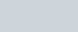So the region around any stationary charged particles, in which if another test charge particles enter then it experiences an electrostatic force is called electric field but what is the region around that charged particles when it is moving with constant acceleration? So here a brand new term added to the physics of moving charges i.e the magnetic field.

If a charge particle is moving with a constant acceleration then it creates a electric field in addition to the magnetic field.

But what will happen when it is not moving with constant acceleration. If it is not moving with constant acceleration, it creates an electric field in addition to the magnetic field and electromagnetic radiation. They radiate energy in the form of electromagnetic radiation.

### DEFINITION

Magnetic field is the three dimensional space or region around any magnetic material or (current carring conductor) in which if another magnetic material tries to enter then it’s experience a magnetic force.

We know that the formula for an electric field can be given as –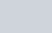where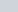is the electric force and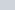is electric charge.

So by following this analogy we can also give the formula for the magnetic field. The magnetic field formula can be given as-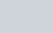whereis magnetic force andis the magnetic charge or magnetic pole strength.

## IMPORTANT POINTS RELATED TO MAGNETIC FIELD

1. The magnetic field in a region is uniform if the magnitude of its strength and its direction is the same at every point in that region.
2. The strength of the magnetic field is sometimes known as magnetic induction and magnetic flux density.
3. The SI unit of the magnetic field is Tesla and it is denoted as capital T.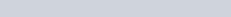i.e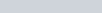. In the form of Weber, it is given as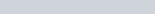i.e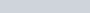4. In the form of gauss (G), it is given as-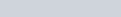## MAGNETIC FIELDS LINES

As an electric field, the magnetic field is also invisible. We can’t tell how the magnetic field looks like. But there are various ways by which we can view the alignment and orientation of magnetic field around any magnetic material.

One of the popular ways in spreading the iron filings around the bar magnet. If you sprinkle some iron filings around the bar magnet then you will see that iron filings arrange themselves in the same way as that of the magnetic field of the bar magnet. See figure below:Fig. 2, Illustration of magnetic field lines of a bar magnet. Source: exploratorium

You can see that the iron filings aligned in the direction of the magnetic field and it forms a curve. What is this curve? This curve is nothing but the magnetic field lines which was introduced by Michael Faraday.

One may ask a question that why they align themselves in the direction of the magnetic field lines? The magnetic field lines are also called magnetic lines of force because they forced the small magnets or other magnetic material to align in the direction of the magnetic field.

Here small iron filings experience a force due to these field lines and this magnetic force tends them to align in the direction of the magnetic field.

### WHAT ARE MAGNETIC FIELD LINES?

#### DEFINITION

Magnetic field lines are nothing but it is the pictorial representation of magnetic field. It is the imaginary line used to show the orientation of the magnetic field.fig. 3, pictorial representation of the magnetic field, source: dowling magnets

### PROPERTIES OF MAGNETIC FIELD LINES

Some properties of magnetic field lines are listed below:

1. A magnetic field line is a vector quantity because it has both magnitude and direction.

2. They always form closed loops with no beginning and no end. You might have thought that they exit from the North pole and enter the South pole. But this is not true, they never end. They close the loops even inside the magnet and reappear at the poles and we think that they exit and enter at the poles.

3. Outside a magnet, magnetic field lines are directed from the north pole to the south pole. But inside the magnet or magnetic material, it is directed from the South pole to the North pole.

4. They are closed and continuous curves.

5. It behaves like stretched elastic rubber string.

6. The magnetic field lines are dense near the poles and it is rarer near the center, due to this a stronger magnetic field lies at the poles as compared to the center where the magnetic field is weak.

7. The field lines never intersect each other because it would imply two directions of the magnetic field at that point which is impossible.

8. Parallel and equidistant field lines represent a uniform magnetic field.
9. Tangent is drawn at any point on the magnetic field line gives the direction of the magnetic field at that point.

10. They leave the North pole and enter the South pole perpendicularly.

11. The magnetic lines of force between the two unlike poles contract longitudinally (attractive in nature) and the magnetic lines of force between the two like poles widen laterally (repulsive in nature).fig. 4, attraction and repulsion of magnetic field lines, source: CK-12 Foundation
12. The region where there is no magnetic field has no magnetic lines there. At the neutral point where the resultant magnetic field is zero then there will be no magnetic lines of force.

Watch this video for more understanding.
Stay tuned with Laws Of Nature for more useful and interesting content.